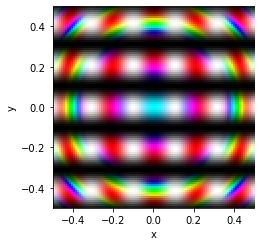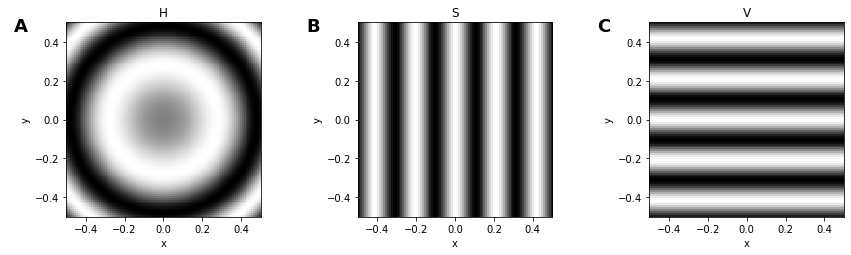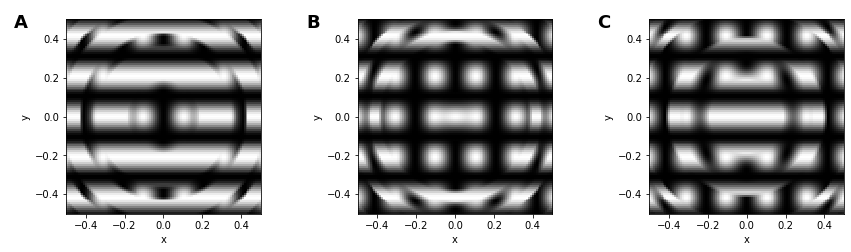# HSV#

Title
HSV Element
Dependencies
Matplotlib
Backends
Matplotlib
Bokeh
```import numpy as np
import holoviews as hv
from holoviews import opts

hv.extension('matplotlib')
```HSV represents a regularly spaced 2D grid of an underlying continuous space of HSV (hue, saturation and value) color space values. The definition of the grid closely matches the semantics of an `Image` or `RGB` element and in the simplest case the grid may be specified as a `NxMx3` or `NxMx4` array of values along with a bounds. An HSV element may also be defined through explicit and regularly spaced x/y-coordinate arrays. The two most basic supported constructors of an HSV element therefore include:

```HSV((X, Y, H, S, V))
```

where `X` is a 1D array of shape `M`, `Y` is a 1D array of shape `N` and `H`/`S`/`V` are 2D array of shape `NxM`, or equivalently:

```HSV(Z, bounds=(x0, y0, x1, y1))
```

where `Z` is a 3D array of stacked `H`/`S`/`V` arrays with shape `NxMx3/4` and the bounds define the (left, bottom, right, top) edges of the four corners of the grid. Other gridded formats which support declaring of explicit x/y-coordinate arrays such as xarray are also supported. See the Gridded Datasets user guide for all the other accepted data formats.

```x,y = np.mgrid[-50:51, -50:51] * 0.1
h = 0.5 + np.sin(0.2*(x**2+y**2)) / 2.0
s = 0.5*np.cos(y*3)+0.5
v = 0.5*np.cos(x*3)+0.5

hsv = hv.HSV(np.dstack([h, s, v]))
hsv
```You can see how this is created from the original channels:

```opts.defaults(opts.Image(cmap='gray'))

hsv[..., 'H'].relabel('H') + hsv[..., 'S'].relabel('S') + hsv[..., 'V'].relabel('V')
```An `HSV` Element can also easily be converted to an `RGB` Element using the `rgb` property:

```print(hsv.rgb)
hsv.rgb[..., 'R'] + hsv.rgb[..., 'G'] + hsv.rgb[..., 'B']
```
```:RGB   [x,y]   (R,G,B)
```For full documentation and the available style and plot options, use `hv.help(hv.HSV).`

This web page was generated from a Jupyter notebook and not all interactivity will work on this website. Right click to download and run locally for full Python-backed interactivity.